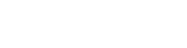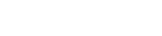Keras、TensorFlow、PyTorch 等高级框架可以帮助我们快速构建复杂模型。深入研究并理解其中的理念很有价值。不久前，本文作者发表了一篇文章（参见《资源 | 来自独秀同学的深度网络数学笔记，还不快收藏？》），简明扼要地解释了神经网络的工作原理，但那篇文章偏向于数学理论知识。所以作者打算以一种更实际的方式来跟进这一话题。他们尝试只使用 构建一个全运算的神经网络，通过解决简单的分类问题来测试模型，并将其与 Keras 构建的神经网络进行性能比较。图 1 ：密集神经网络架构图 2 ：神经网络框图图 3 ：l 层的权值矩阵 W 和偏置向量 b 的维数。

``````nn_architecture = [
{"input_dim": 2, "output_dim": 4, "activation": "relu"},
{"input_dim": 4, "output_dim": 6, "activation": "relu"},
{"input_dim": 6, "output_dim": 6, "activation": "relu"},
{"input_dim": 6, "output_dim": 4, "activation": "relu"},
{"input_dim": 4, "output_dim": 1, "activation": "sigmoid"},
]
``````

Snippet 1：包含描述特定神经网络参数的列表。该列表对应图 1 所示的 NN。

``````def init_layers(nn_architecture, seed = 99):
np.random.seed(seed)
number_of_layers = len(nn_architecture)
params_values = {}
for idx, layer in enumerate(nn_architecture):
layer_idx = idx + 1
layer_input_size = layer["input_dim"]
layer_output_size = layer["output_dim"]
params_values['W' + str(layer_idx)] = np.random.randn(
layer_output_size, layer_input_size) * 0.1
params_values['b' + str(layer_idx)] = np.random.randn(
layer_output_size, 1) * 0.1
return params_values
``````

Snippet 2：初始化权值矩阵和偏置向量值的函数。图 4：算法中使用的激活函数

``````def sigmoid(Z):
return 1/(1+np.exp(-Z))
def relu(Z):
return np.maximum(0,Z)
def sigmoid_backward(dA, Z):
sig = sigmoid(Z)
return dA * sig * (1 - sig)
def relu_backward(dA, Z):
dZ = np.array(dA, copy = True)
dZ[Z <= 0] = 0;
return dZ;
``````

Snippet 3：ReLU 和 Sigmoid 激活函数及其导数。

``````def single_layer_forward_propagation(A_prev, W_curr, b_curr, activation="relu"):
Z_curr = np.dot(W_curr, A_prev) + b_curr
if activation is "relu":
activation_func = relu
elif activation is "sigmoid":
activation_func = sigmoid
else:
raise Exception('Non-supported activation function')
return activation_func(Z_curr), Z_curr
``````

Snippet 4：单层前向传播步骤图 5 ：在前向传播中使用的单个矩阵的维数。

``````def full_forward_propagation(X, params_values, nn_architecture):
memory = {}
A_curr = X
for idx, layer in enumerate(nn_architecture):
layer_idx = idx + 1
A_prev = A_curr
activ_function_curr = layer["activation"]
W_curr = params_values["W" + str(layer_idx)]
b_curr = params_values["b" + str(layer_idx)]
A_curr, Z_curr = single_layer_forward_propagation(A_prev, W_curr, b_curr, activ_function_curr)
memory["A" + str(idx)] = A_prev
memory["Z" + str(layer_idx)] = Z_curr
return A_curr, memory
``````

Snippnet 5：完整前向传播步骤``````def get_cost_value(Y_hat, Y):
m = Y_hat.shape
cost = -1 / m * (np.dot(Y, np.log(Y_hat).T) + np.dot(1 - Y, np.log(1 - Y_hat).T))
return np.squeeze(cost)
def get_accuracy_value(Y_hat, Y):
Y_hat_ = convert_prob_into_class(Y_hat)
return (Y_hat_ == Y).all(axis=0).mean()
``````

Snippnet 6：损失函数准确率计算

``````def single_layer_backward_propagation(dA_curr, W_curr, b_curr, Z_curr, A_prev, activation="relu"):
m = A_prev.shape
if activation is "relu":
backward_activation_func = relu_backward
elif activation is "sigmoid":
backward_activation_func = sigmoid_backward
else:
raise Exception('Non-supported activation function')
dZ_curr = backward_activation_func(dA_curr, Z_curr)
dW_curr = np.dot(dZ_curr, A_prev.T) / m
db_curr = np.sum(dZ_curr, axis=1, keepdims=True) / m
dA_prev = np.dot(W_curr.T, dZ_curr)
return dA_prev, dW_curr, db_curr
``````

Snippnet 7：单层反向传播步骤图 6：一层中的前向和反向传播。``````def full_backward_propagation(Y_hat, Y, memory, params_values, nn_architecture):
m = Y.shape
Y = Y.reshape(Y_hat.shape)
dA_prev = - (np.divide(Y, Y_hat) - np.divide(1 - Y, 1 - Y_hat));
for layer_idx_prev, layer in reversed(list(enumerate(nn_architecture))):
layer_idx_curr = layer_idx_prev + 1
activ_function_curr = layer["activation"]
dA_curr = dA_prev
A_prev = memory["A" + str(layer_idx_prev)]
Z_curr = memory["Z" + str(layer_idx_curr)]
W_curr = params_values["W" + str(layer_idx_curr)]
b_curr = params_values["b" + str(layer_idx_curr)]
dA_prev, dW_curr, db_curr = single_layer_backward_propagation(
dA_curr, W_curr, b_curr, Z_curr, A_prev, activ_function_curr)
``````

Snippnet 8：全反向传播步骤``````def update(params_values, grads_values, nn_architecture, learning_rate):
for layer_idx, layer in enumerate(nn_architecture):
params_values["W" + str(layer_idx)] -= learning_rate * grads_values["dW" + str(layer_idx)]
params_values["b" + str(layer_idx)] -= learning_rate * grads_values["db" + str(layer_idx)]
return params_values;
``````

Snippnet 9：利用梯度下降更新参数

``````def train(X, Y, nn_architecture, epochs, learning_rate):
params_values = init_layers(nn_architecture, 2)
cost_history = []
accuracy_history = []
for i in range(epochs):
Y_hat, cashe = full_forward_propagation(X, params_values, nn_architecture)
cost = get_cost_value(Y_hat, Y)
cost_history.append(cost)
accuracy = get_accuracy_value(Y_hat, Y)
accuracy_history.append(accuracy)
grads_values = full_backward_propagation(Y_hat, Y, cashe, params_values, nn_architecture)
params_values = update(params_values, grads_values, nn_architecture, learning_rate)
return params_values, cost_history, accuracy_history
``````

Snippnet 10：训练模型
David vs Goliath图 7：测试数据集图 8：两种模型实现的分类边界可视化